• 超像素分割为图像分割，图像处理的基础，文件为MATLAB和C混编的代码，demo为主程序，运行demo主程序进行超像素分割
• 本代码是论文: Liu M Y, Tuzel O, Ramalingam S, et al. Entropy rate superpixel segmentation[C]// Computer Vision and Pattern Recognition. IEEE, 2011:2097-2104. 的实现代码，网络上的原始链接均已失效，分享...
• 超像素分割算法，亲测可用，内含数据图片，不用mex，直接matlab运行
• 简介：最近项目使用到了超像素分割，因此顺道研究了以下SLIC这一算法超像素分割这类low-level vision问题已经在CVPR，ICCV这种顶级会议上逐渐销声匿迹，越来越流行的learning method渐渐占据了这些顶级会议90%的...

简介：最近项目使用到了超像素分割，因此顺道研究了以下SLIC这一算法。超像素分割这类low-level vision问题已经在CVPR，ICCV这种顶级会议上逐渐销声匿迹，越来越流行的learning method渐渐占据了这些顶级会议90%的篇幅。本文讲解的SLIC是2010年提出的一种十分简单的超分辨分割算法，原理简单、便于实现。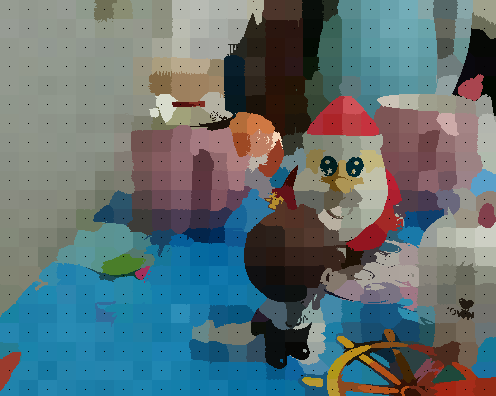一.SLIC(simple linear iterative clustering）原理分析

1. 初始化种子点（聚类中心）：按照设定的超像素个数，在图像内均匀的分配种子点。假设图片总共有 N 个像素点，预分割为 K 个相同尺寸的超像素，那么每个超像素的大小为N/ K ，则相邻种子点的距离（步长）近似为S=sqrt(N/K)。

2. 在种子点的n*n邻域内重新选择种子点（一般取n=3）。具体方法为：计算该邻域内所有像素点的梯度值，将种子点移到该邻域内梯度最小的地方。这样做的目的是为了避免种子点落在梯度较大的轮廓边界上，以免影响后续聚类效果。

3. 在每个种子点周围的邻域内为每个像素点分配类标签（即属于哪个聚类中心）。和标准的k-means在整张图中搜索不同，SLIC的搜索范围限制为2S2S，可以加速算法收敛，如下图。在此注意一点：期望的超像素尺寸为SS，但是搜索的范围是2S*2S。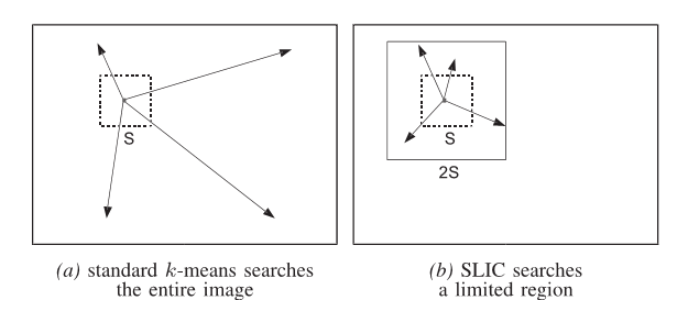4. 距离度量。包括颜色距离和空间距离。对于每个搜索到的像素点，分别计算它和该种子点的距离。距离计算方法如下：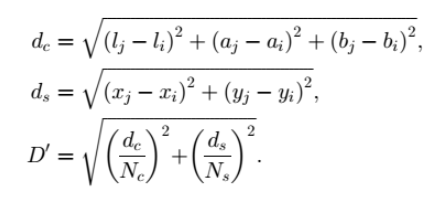其中，dc代表颜色距离，ds代表空间距离，Ns是类内最大空间距离，定义为Ns=S=sqrt(N/K)，适用于每个聚类。最大的颜色距离Nc既随图片不同而不同，也随聚类不同而不同，所以我们取一个固定常数m（取值范围[1,40],一般取10）代替。最终的距离度量D’如下：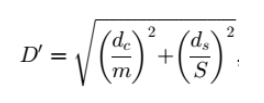由于每个像素点都会被多个种子点搜索到，所以每个像素点都会有一个与周围种子点的距离，取最小值对应的种子点作为该像素点的聚类中心。

1. 迭代优化。理论上上述步骤不断迭代直到误差收敛（可以理解为每个像素点聚类中心不再发生变化为止），实践发现10次迭代对绝大部分图片都可以得到较理想效果，所以一般迭代次数取10。

2. 增强连通性。经过上述迭代优化可能出现以下瑕疵：出现多连通情况、超像素尺寸过小，单个超像素被切割成多个不连续超像素等，这些情况可以通过增强连通性解决。主要思路是：新建一张标记表，表内元素均为-1，按照“Z”型走向（从左到右，从上到下顺序）将不连续的超像素、尺寸过小超像素重新分配给邻近的超像素，遍历过的像素点分配给相应的标签，直到所有点遍历完毕为止。

二.伪算法描述

/∗ Initialization ∗/
Initialize cluster centers Ck = [lk , ak , bk , xk , yk ]T by sampling pixels at regular grid steps S.
Move cluster centers to the lowest gradient position in a 3 × 3 neighborhood.
Set label l(i) =1 for each pixel i. Set distance d(i) =for each pixel i.

repeat
/∗ Assignment ∗/
for each cluster center Ck do
for each pixel i in a 2S × 2S region around Ck do
Compute the distance D between Ck and i.
if D < d(i) then
set d(i) = D
set l(i) = k
end if
end for
end for

/∗ Update ∗/
Compute new cluster centers. Compute residual error E.
until E ≤ threshold

三.参考博文

展开全文超像素 图像处理 深度学习 python
• 写完这篇，图像分割的传统方法就快全了，传统图像分割大体有基于阈值的，这类就没啥算法可以写，所以直接略过了；...最后就是超像素分割了，超像素分割有k-means算法的影子，所以可以先看看k-means算法的代码实现过程.写完这篇，图像分割的传统方法就快全了，传统图像分割大体有基于阈值的，这类就没啥算法可以写，所以直接略过了；然后就是K-means这种聚类/分裂的，从几个点开始进行聚类分割，或者一张图不断分裂达到分割目的；

【手撕算法】K-means算法实现主题色提取

再有就是区域生长这类的；

【手撕算法】基于队列实现的区域增长分割算法

以及分水岭算法，分水岭算法代码写好有一段时间了，但实在不知道文章咋写…就再放放吧；最后就是超像素分割了，超像素分割有k-means算法的影子，所以可以先看看k-means算法的代码实现过程。

1，算法原理

1. 初始化种子点（聚类中心）：按照设定的超像素个数，在图像内均匀的分配种子点。假设图片总共有 N 个像素点，预分割为 K 个相同尺寸的超像素，那么每个超像素的大小为N/ K ，则相邻种子点的距离（步长）近似为S=sqrt(N/K)。

2. 在种子点的n*n邻域内重新选择种子点（一般取n=3）。具体方法为：计算该邻域内所有像素点的梯度值，将种子点移到该邻域内梯度最小的地方。这样做的目的是为了避免种子点落在梯度较大的轮廓边界上，以免影响后续聚类效果。

3. 在每个种子点周围的邻域内为每个像素点分配类标签（即属于哪个聚类中心）。和标准的k-means在整张图中搜索不同，SLIC的搜索范围限制为2S2S，可以加速算法收敛，如下图。在此注意一点：期望的超像素尺寸为SS，但是搜索的范围是2S*2S。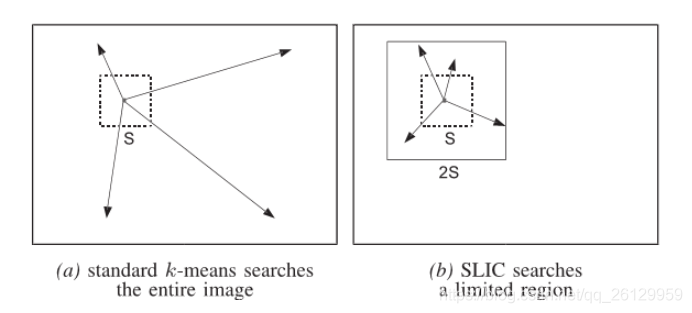4. 距离度量。包括颜色距离和空间距离。对于每个搜索到的像素点，分别计算它和该种子点的距离。距离计算方法如下：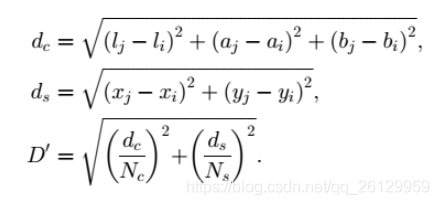其中，dc代表颜色距离，ds代表空间距离，Ns是类内最大空间距离，定义为Ns=S=sqrt(N/K)，适用于每个聚类。最大的颜色距离Nc既随图片不同而不同，也随聚类不同而不同，所以我们取一个固定常数m（取值范围[1,40],一般取10）代替。最终的距离度量D’如下：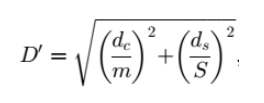由于每个像素点都会被多个种子点搜索到，所以每个像素点都会有一个与周围种子点的距离，取最小值对应的种子点作为该像素点的聚类中心。

5. 迭代优化。理论上上述步骤不断迭代直到误差收敛（可以理解为每个像素点聚类中心不再发生变化为止），实践发现10次迭代对绝大部分图片都可以得到较理想效果，所以一般迭代次数取10。

6. 增强连通性。经过上述迭代优化可能出现以下瑕疵：出现多连通情况、超像素尺寸过小，单个超像素被切割成多个不连续超像素等，这些情况可以通过增强连通性解决。主要思路是：新建一张标记表，表内元素均为-1，按照“Z”型走向（从左到右，从上到下顺序）将不连续的超像素、尺寸过小超像素重新分配给邻近的超像素，遍历过的像素点分配给相应的标签，直到所有点遍历完毕为止。

2，伪算法描述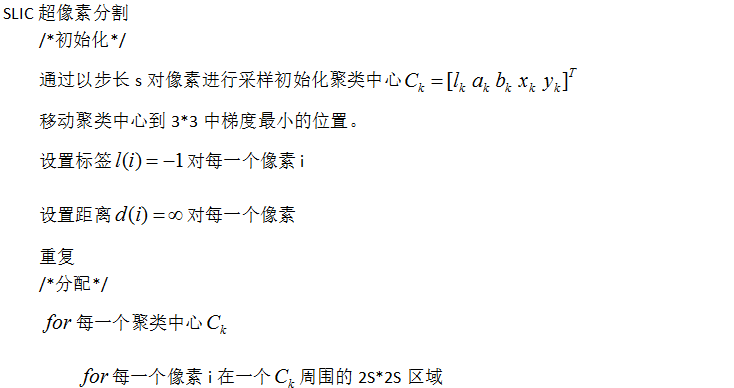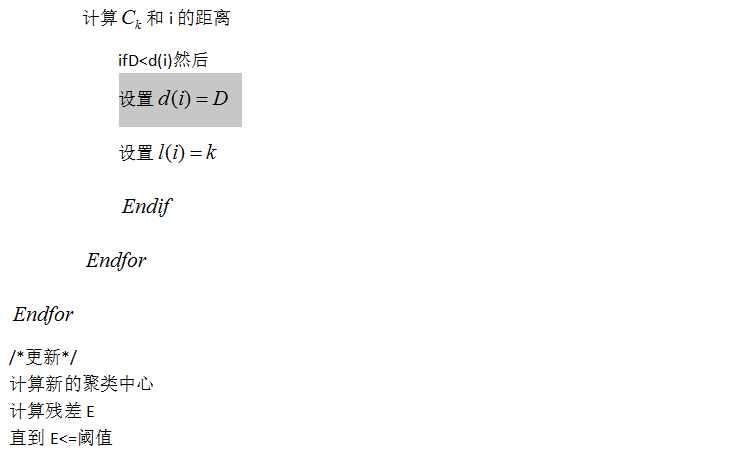3，程序介绍

程序声明了一个SLIC算法类，类的具体程序太长了，就不贴了，大家可以去qq群【222954293】下载程序自己看，都注释好了。

就看一下主程序吧：

int main()
{
【1】读取原图并显示
if (image.empty())
{
printf_s("图片读取失败");
return -1;
}
imshow("原图", image);
【2】转换为LAB颜色空间  方便计算距离
Mat lab_image = image.clone();
cvtColor(image, lab_image, COLOR_BGR2Lab);

//定义超像素数以及权重
int w = image.cols, h = image.rows;
int nr_superpixels = 300;//超像素数
int nc = 40;//权重m
double step = sqrt((w * h) / (double)nr_superpixels);
【3】执行SLIC超像素算法
SLIC slic;
slic.generate_superpixels(&lab_image, step, nc);
slic.create_connectivity(&lab_image);

【4】显示分割轮廓和分割结果图
//该三个函数可以分别注释单独显示查看
slic.colour_with_cluster_means(&image);//颜色均值填充
slic.display_contours(&image, Scalar(0, 0, 255));//显示轮廓
//slic.display_center_grid(&image, Scalar(255, 0, 0));//显示中心点
imshow("result", image);
waitKey(0);
}

一共是四个步骤。其中步骤【2】中需要自己定义两个变量nr_superpixels和nc。

• nr_superpixels为超像素个数，你可以根据图像大小自己定义，如果图像x方向10个超像素块，y方向30个超像素块，那就是300。
• 权重变量nc，即上文【算法原理】第4步中的固定常数m，一般取1-40范围内的整数。

3，效果展示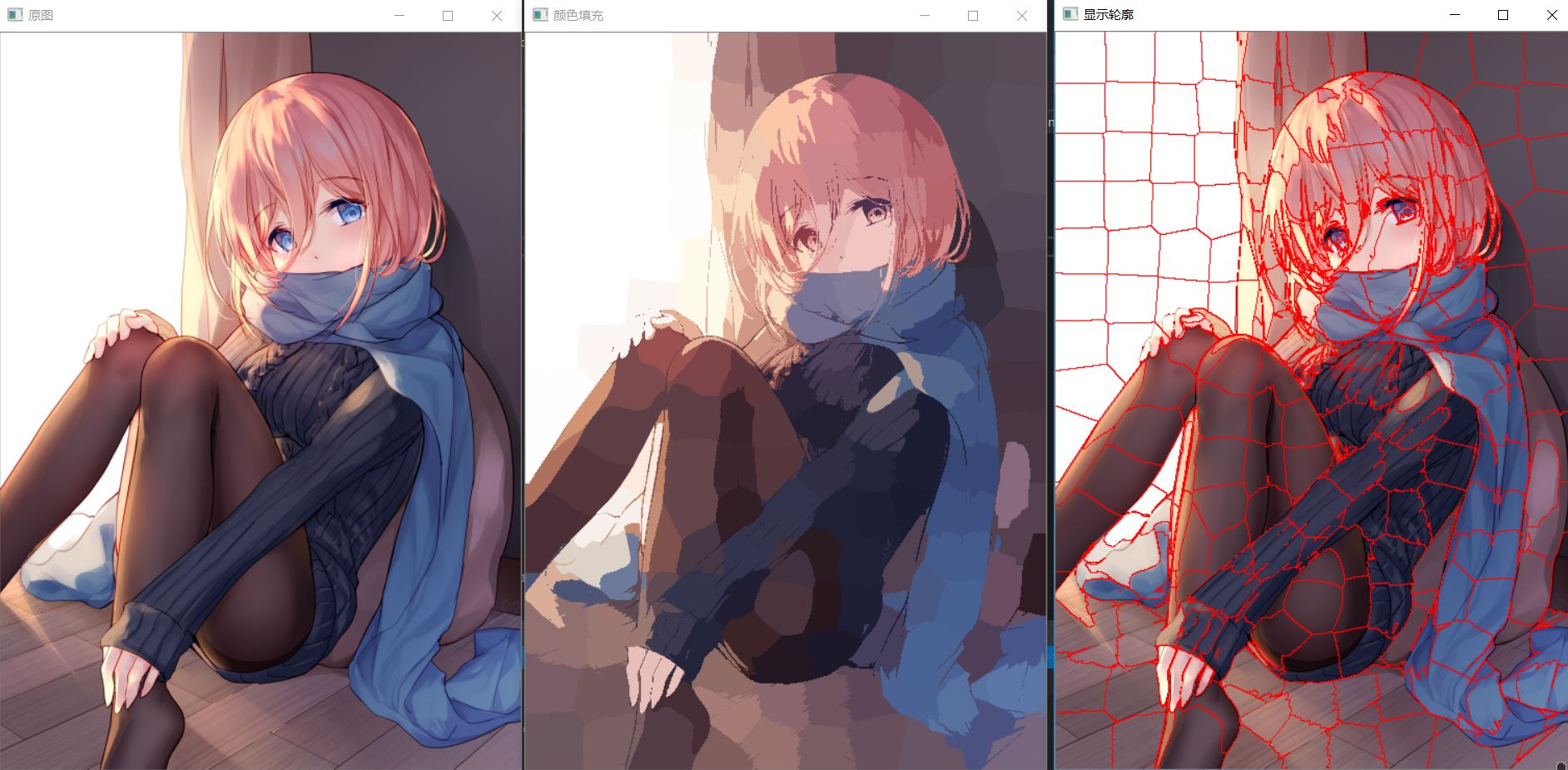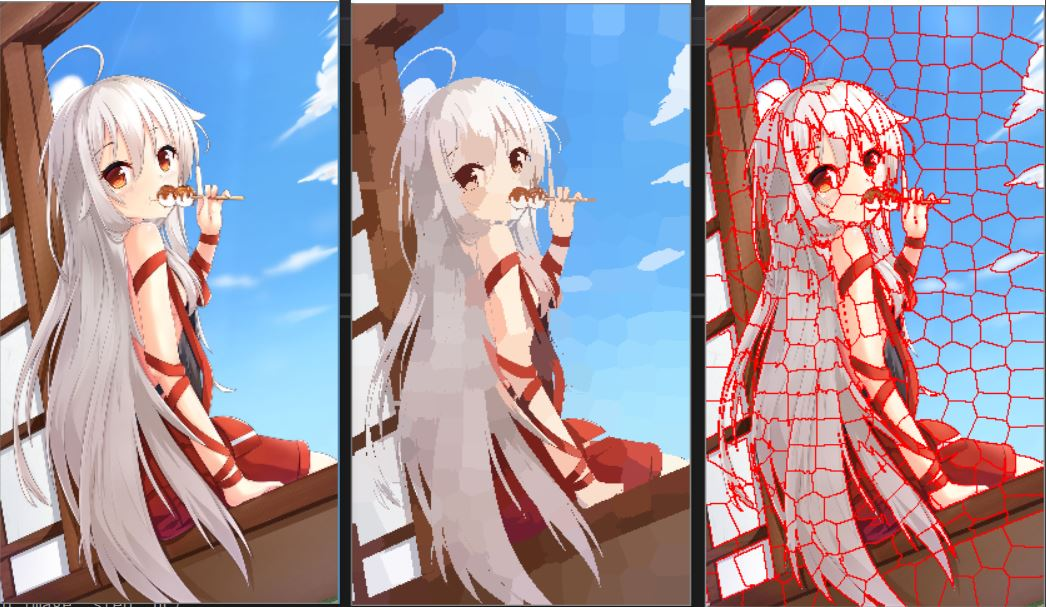4，THE END

本文原创内容有限，就是整合了一下自己看的超像素分割的博客，两篇不错的链接放这儿了：

https://blog.csdn.net/zhj_matlab/article/details/52986700
https://blog.csdn.net/qq_26129959/article/details/90760028

代码放qq群【222954293】了，今天就到这里啦。

展开全文算法 聚类 opencv 图像处理 超像素分割
• Python实现超像素分割 图像分割：Python的SLIC超像素分割 二、SLIC库的介绍 python 自带slic代码分析 一句代码 高层API python 使用SLIC 超像素分割 三、SLIC库的使用 SLIC超像素分割并保存分割得到的超像素块...
展开全文算法 python 机器学习
• 超像素分割算法SLIC的matlab实现

千次阅读 多人点赞 2020-08-20 16:59:05
SLIC是一种基于网格化KMeans聚类的超像素分割算法，其计算复杂度为O(N)，其中N为像素点个数。SLIC的原理比较简单精致，具体的原理我这里就不介绍了，推荐大家自己去读原始论文加深理解。SLIC的算法流程如下： 如...

SLIC是一种基于网格化KMeans聚类的超像素分割算法，其计算复杂度为O(N)，其中N为像素点个数。SLIC的原理比较简单精致，具体的原理我这里就不介绍了，推荐大家自己去读原始论文加深理解（但我以为看下面这个算法流程图就足够理解原理了）。SLIC的算法流程如下：如所有其他聚类算法一样，SLIC不能保证连通性，所以需要后处理将旁生的连通域合并到邻近的主连通域上，但是论文中并未给出具体的后处理方法。我给出的方法是按照轮廓接触点个数最多原则合并连通域。由于每个聚类都有自己的“势力范围”，即每个标签覆盖的区域不会超过聚类时限定的范围（一般是2S*2S大小，边缘栅格的聚类例外），所以合并处理时只需要在该范围内操作即可。

下面给出本人实现的SLIC算法程序（控制色域与空域权重比例的系数wDs应设为函数形参，这里就不改了。注意！迭代中限定聚类的栅格是不变的！！！）：

function Label=SLIC(img,s,errTh,wDs)
% 基于KMeans的超像素分割
% img为输入图像，维度不限，最大值为255
% s x s为超像素尺寸
% errTh为控制迭代结束的联合向量残差上限
m=size(img,1);
n=size(img,2);

%% 计算栅格顶点与中心的坐标
h=floor(m/s);
w=floor(n/s);
rowR=floor((m-h*s)/2); %多余部分首尾均分
colR=floor((n-w*s)/2);
rowStart=(rowR+1):s:(m-s+1);
rowStart(1)=1;
rowEnd=rowStart+s;
rowEnd(1)=rowR+s;
rowEnd(end)=m;
colStart=(colR+1):s:(n-s+1);
colStart(1)=1;
colEnd=colStart+s;
colEnd(1)=colR+s;
colEnd(end)=n;
rowC=floor((rowStart+rowEnd-1)/2);
colC=floor((colStart+colEnd-1)/2);
% 显示划分结果
temp=zeros(m,n);
temp(rowStart,:)=1;
temp(:,colStart)=1;
for i=1:h
for j=1:w
temp(rowC(i),colC(j))=1;
end
end
figure,imshow(temp);
imwrite(temp,'栅格.bmp');

%% 计算梯度图像，使用sobel算子和欧式距离
img=double(img)/255;
r=img(:,:,1);
g=img(:,:,2);
b=img(:,:,3);
Y=0.299 * r + 0.587 * g + 0.114 * b;

f1=fspecial('sobel');
f2=f1';
gx=imfilter(Y,f1);
gy=imfilter(Y,f2);
G=sqrt(gx.^2+gy.^2);

%% 选择栅格中心点3*3邻域中梯度最小点作为起始点
rowC_std=repmat(rowC',[1,w]);
colC_std=repmat(colC,[h,1]);
rowC=rowC_std;
colC=colC_std;
for i=1:h
for j=1:w
block=G(rowC(i,j)-1:rowC(i,j)+1,colC(i,j)-1:colC(i,j)+1);
[minVal,idxArr]=min(block(:));
jOffset=floor((idxArr(1)+2)/3);
iOffset=idxArr(1)-3*(jOffset-1);
rowC(i,j)=rowC(i,j)+iOffset;
colC(i,j)=colC(i,j)+jOffset;
end
end

%% KMeans超像素分割
Label=zeros(m,n)-1;
dis=Inf*ones(m,n);
M=reshape(img,m*n,size(img,3)); %像素值重排
% 联合色域值和空域值
colorC=zeros(h,w,size(img,3));
for i=1:h
for j=1:w
colorC(i,j,:)=img(rowC(i),colC(j),:);
end
end
uniMat=cat(3,colorC,rowC,colC);
uniMat=reshape(uniMat,h*w,size(img,3)+2);
iter=1;
while(1)
uniMat_old=uniMat;
%     rowC_old=rowC;
%     colC_old=colC;
for k=1:h*w
c=floor((k-1)/h)+1;
r=k-h*(c-1);
rowCidx=rowC(r,c);
colCidx=colC(r,c); %聚类中心坐标
%聚类限定的栅格(中心点始终是原s x s栅格的中心点)
rowStart=max(1,rowC_std(r,c)-s);
rowEnd=min(m,rowC_std(r,c)+s-1);
colStart=max(1,colC_std(r,c)-s);
colEnd=min(n,colC_std(r,c)+s);
%         colorC=uniMat(k,1:size(img,3));
colorC=M((colCidx-1)*m+rowCidx,:);
for i=rowStart:rowEnd
for j=colStart:colEnd
colorCur=M((j-1)*m+i,:);
dc=norm(colorC-colorCur);
ds=norm([i-rowCidx,j-colCidx]);
d=dc^2+wDs*(ds/s)^2;
if d<dis(i,j)
dis(i,j)=d;
Label(i,j)=k;
end
end
end
end

%显示聚类结果
temp=mod(Label,20)+1;
figure;
imagesc(label2rgb(temp-1,'jet','w','shuffle')) ;
axis image ; axis off ;
% 录制gif
F=getframe(gcf);
I=frame2im(F);
[I,map]=rgb2ind(I,256);
if iter == 1
imwrite(I,map,'test.gif','gif','Loopcount',inf,'DelayTime',0.2);
else
imwrite(I,map,'test.gif','gif','WriteMode','append','DelayTime',0.2);
end
iter=iter+1;

% 更新聚类中心
colorC=zeros(h,w,size(img,3));
for k=1:h*w
num=0;
sumColor=zeros(1,size(img,3));
sumR=0;
sumC=0;
c=floor((k-1)/h)+1;
r=k-h*(c-1);
rowCidx=rowC_std(r,c);
colCidx=colC_std(r,c);
rowStart=max(1,rowCidx-s);
rowEnd=min(m,rowCidx+s-1);
colStart=max(1,colCidx-s);
colEnd=min(n,colCidx+s);

for row=rowStart:rowEnd
for col=colStart:colEnd
if Label(row,col)==k
num=num+1;
sumR=sumR+row;
sumC=sumC+col;
color=reshape(img(row,col,:),1,size(img,3));
sumColor=sumColor+color;
end
end
end
colorC(r,c,:)=sumColor/num;
rowC(r,c)=round(sumR/num);
colC(r,c)=round(sumC/num);
end
uniMat=cat(3,colorC,rowC,colC);
uniMat=reshape(uniMat,h*w,size(img,3)+2);
diff=uniMat-uniMat_old;
diff(:,1:2)=sqrt(wDs)*diff(:,1:2)/s;
err=norm(diff)/sqrt(h*w);
if err<errTh %残差低于阈值，结束迭代
break;
end
end

%% 后处理， 按照边界接触点数最多原则分配小连通域的标签
for k=1:h*w
c=floor((k-1)/h)+1;
r=k-h*(c-1);
rowCidx=rowC_std(r,c);
colCidx=colC_std(r,c);
rowStart=max(1,rowCidx-s);
rowEnd=min(m,rowCidx+s-1);
colStart=max(1,colCidx-s);
colEnd=min(n,colCidx+s);
block=Label(rowStart:rowEnd,colStart:colEnd);
block(block~=k)=0;
block(block==k)=1;
label=bwlabel(block);
szlabel=max(label(:)); %标签个数
bh=rowEnd-rowStart+1;
bw=colEnd-colStart+1;  %block的宽高

if szlabel<2  %无伴生连通域，略过
continue;
end

labelC=label(rowCidx-rowStart+1,colCidx-colStart+1); %主连通域的标记值
top=max(1,rowStart-1);
bottom=min(m,rowEnd+1);
left=max(1,colStart-1);
right=min(n,colEnd+1);
for i=1:szlabel %遍历连通域
if i==labelC %主连通域不处理
continue;
end
marker=zeros(bottom-top+1,right-left+1); %生成一个外扩一圈的marker，标记哪些点已经被统计过接触情况
bw=label;
bw(bw~=i)=0;
bw(bw==i)=1; %当前连通域标记图
contourBW=bwperim(bw); %求取外轮廓
%             figure,imshow(contourBW);
idxArr=find(double(contourBW)==1);
labelArr=zeros(4*length(idxArr),1);  %记录轮廓点的4邻域点标记值的向量
num=0;
for idx=1:size(idxArr) %遍历轮廓点,统计其4邻域点的标记值
bc=floor((idxArr(idx)-1)/bh)+1;
br=idxArr(idx)-bh*(bc-1); %轮廓点在block中的行列信息
row=br+rowStart-1;
col=bc+colStart-1; %轮廓点在大图中的行列信息
rc=[row-1,col;...
row+1,col;...
row,col-1;...
row,col+1];
for p=1:4
row=rc(p,1);
col=rc(p,2);

if ~(row>=1 && row<=m && col>=1 && col<=n && Label(row,col)~=k)
continue;
end

if marker(row-top+1,col-left+1)==0 %未被统计过
marker(row-top+1,col-left+1)=1;
num=num+1;
labelArr(num)=Label(row,col);
end
end
end

labelArr(find(labelArr==0))=[]; %去除零元素
uniqueLabel=unique(labelArr);
numArr=zeros(length(uniqueLabel),1);
for p=1:length(uniqueLabel)
idx=find(labelArr==uniqueLabel(p));
numArr(p)=length(idx);
end
idx=find(numArr==max(numArr));
maxnumLabel=uniqueLabel(idx(1)); %接触最多的标签

for row=rowStart:rowEnd
for col=colStart:colEnd
if bw(row-rowStart+1,col-colStart+1)==0
continue;
end
Label(row,col)=maxnumLabel;
end
end
end
end

% 显示连通域处理后聚类结果
temp=mod(Label,20)+1;
figure;
imagesc(label2rgb(temp-1,'jet','w','shuffle')) ;
axis image ; axis off ;

脚本文件：

close all;clc;
figure,imshow(I);

s=15;
errTh=10^-2;
wDs=0.5^2;
Label=SLIC(I,s,errTh,wDs);

%% 显示轮廓
marker=zeros(size(Label));
[m,n]=size(Label);
for i=1:m
for j=1:n
top=Label(max(1,i-1),j);
bottom=Label(min(m,i+1),j);
left=Label(i,max(1,j-1));
right=Label(i,min(n,j+1));
if ~(top==bottom && bottom==left && left==right)
marker(i,j)=1;
end
end
end
figure,imshow(marker);

I2=I;
for i=1:m
for j=1:n
if marker(i,j)==1
I2(i,j,:)=0;
end
end
end
figure,imshow(I2);

测试图像：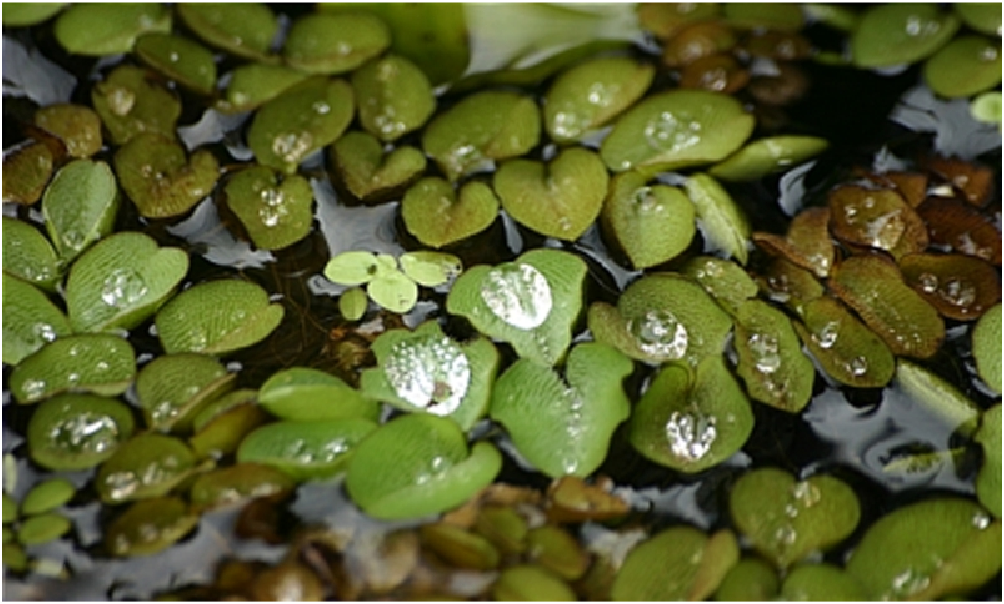栅格划分结果：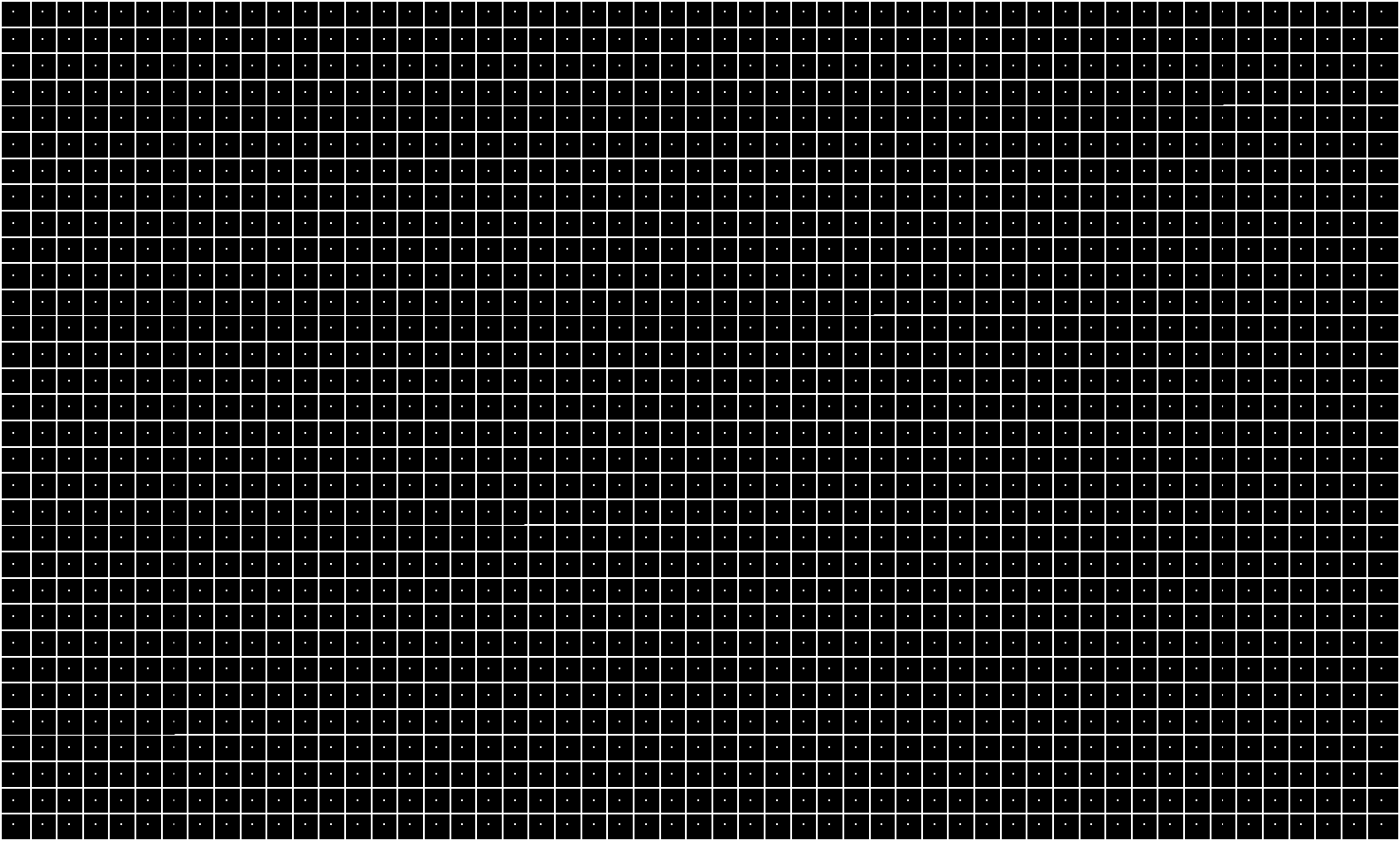聚类过程：聚类最终结果：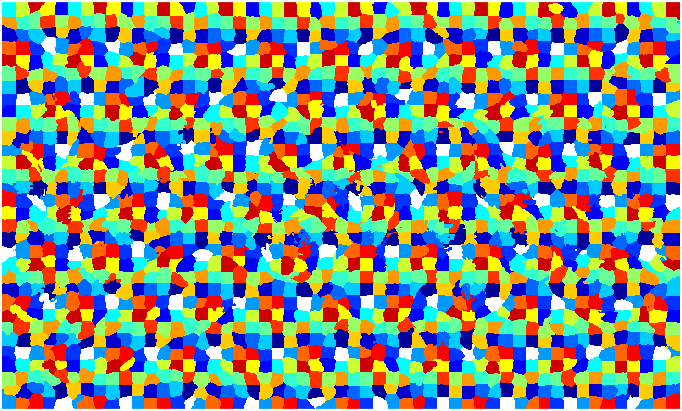连通域合并后的结果：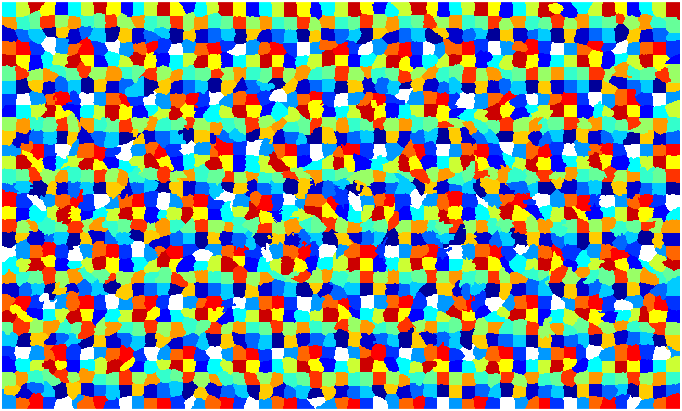原图+轮廓线：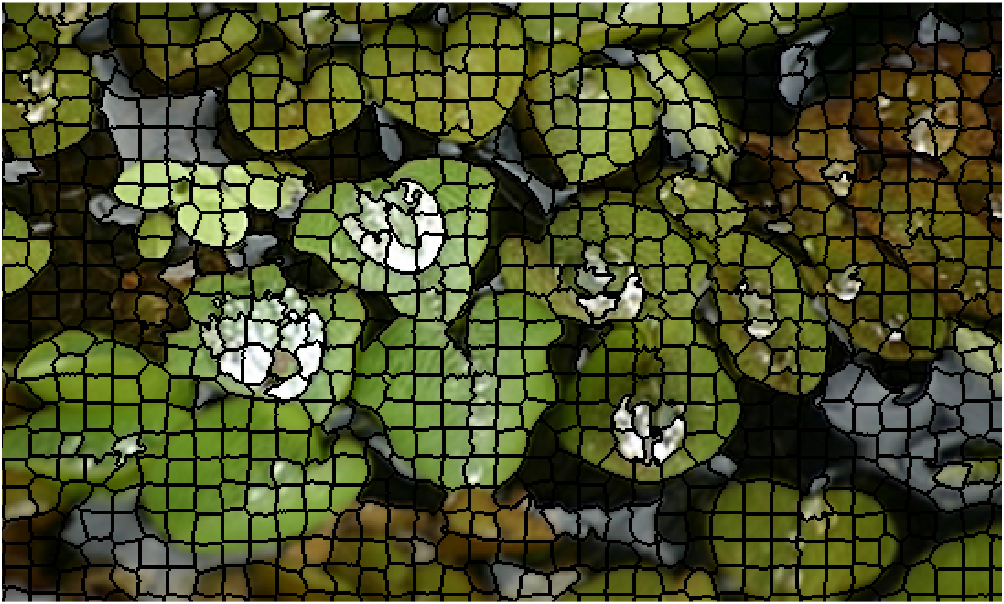具体的我就不解释了，自觉程序写得还是很有条理的，读者自己跟踪程序运行进行理解吧。

展开全文• 亲测可用的SRM分割算法，可用于图像分割（超像素分割
• SLIC超像素分割算法

万次阅读 多人点赞 2017-09-22 18:17:13
超像素概念是2003年Xiaofeng Ren提出和发展起来的图像分割技术，是指具有相似纹理、颜色、亮度等特征的相邻像素构成的有一定视觉意义的不规则像素块。它利用像素之间特征的相似性将像素分组...几种常见的超像素分割方法超像素分割算法
• 超像素能够捕获图像冗余信息，降低后续处理任务复杂度，已受到了国内外研究者的...在此基础上，就目前常用的超像素分割算法进行了实验对比，分析各自的优势和不足。最后，对超像素分割技术的最新应用进行了介绍和展望。超像素 图像分割 梯度下降
•超像素分割
• 超像素分割算法

千次阅读 2015-05-21 10:29:07
在迭代结束时，会有少量的迷失像素，即少量的像素和附近较大的分割块有相同的 标签，但它们并没有被关联进去。因为迭代聚类时没有明确地加强连通性，这种情况就可能发生。因此，我们可以在算法
• 对比了多种超像素算法的边缘召回率、欠分割错误率和紧凑度等评价指标，分析了各自的优势和不足。当前的超像素方法在精度和效率上都有较大的提高，应用领域不断增加，但仍难以满足特殊应用领域的超像素性能要求，需要...
• 超像素能够捕获图像冗余信息，降低后续处理任务复杂度，已受到了国内外研究者的...在此基础上，就目前常用的超像素分割算法进行了实验对比，分析各自的优势和不足。最后， 对超像素分割技术的最新应用进行了介绍和展望
• 文件中包括代码，测试图片，以及代码使用的详细方法。
• 介绍SLIC超像素分割算法，给出其与openCV的接口，代码用VS2012和openCV2.4.9测试可运行。opencv
• SILC算法详解一、原理介绍SLIC算法是simple linear iterative cluster的简称，该算法用来生成超像素(superpixel)算法步骤：已知一副图像大小M*N,可以从RGB空间转换为LAB空间，LAB颜色空间表现的颜色更全面假如预定义...
• 题目如下： 简单解法（HSV 直方图阈值）如下： ...这一节先讲SLIC超像素算法，下一节讲在超像素基础上用Kmeans分类进行分割，参考博客如下： https://www.jianshu.com/p/d0ef931b3ddf https://blog.csdn.net/duyue...opencv c++ SLIC 超像素
• 融合FPGA技术的改进SLIC超像素分割算法.pdf
• OpenCV3中超像素分割算法SEEDS，SLIC， LSC算法在Contrib包里，需要使用Cmake编译使用。为了方便起见，我将三种算法的源码文件从contrib包里拎了出来，可以直接使用，顺便比较一下算法的效果。 　三种算法的源码...
• 超像素-SLIC 简单线性迭代聚类 (SLIC) 超像素分割算法的 OpenCV 实现 用法 有关示例使用，请参阅 main.cpp。 样本输出 原图 计算出的超像素边界 重新着色 参考 原始论文可以在 找到C++
• superpixels-segmentation-gui-opencv：带有QT和OpenCV超像素分割算法，带有漂亮的GUI可以使单元着色
• 源代码为C代码、需编译成MATLAB可执行文件后使用。经本人使用验证有效。。有问题的可以私信。。该代码确定可以实现相应的功能。。SLIC
• 使用简单线性迭代聚类(SLIC)算法对遥感影像进行超像素分割时,存在运行时间长与边缘贴合度差的问题,因此,提出了一种基于改进SLIC的遥感图像超像素分割算法。首先,改进了初始种子点的初始化方式,消除了随机分配造成的......